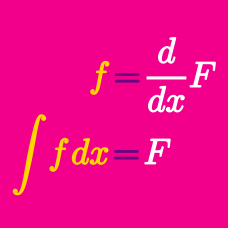Calculus

# Antiderivatives: Level 2 Challenges

$\large \int \sin (x) \, dx - \int \sin (x ) \, dx = \ ?$

Determine the indefinite integral of the following expression: $(\tan{x}) ( \tan{x}+ \sec{x} ).$

Details and Assumptions:

Use $C$ as the constant of the integration.

If $f(x)=\displaystyle \int f(x)dx$,

then $\displaystyle \int \left( f''(x)+(f'(x))^2+(f(x))^3 \right)dx=0$ will give $\displaystyle \sum_{r=1}^3 \dfrac{(f(x))^r}{r}=?$

If $f\left( \frac{3x-4}{3x+4} \right) = x + 2$, then what is the antiderivative of $f(x)$?

Clarification: Take $C$ as an arbitrary constant.

If an antiderivative of $f\left( x \right)$ is ${ e }^{ x }$ and that of $g\left( x \right)$ is $\cos { x }$, then $\displaystyle \int { f\left( x \right) \cos { x } \ dx } +\int { g\left( x \right) { e }^{ x } \ dx }$ is equal to

×